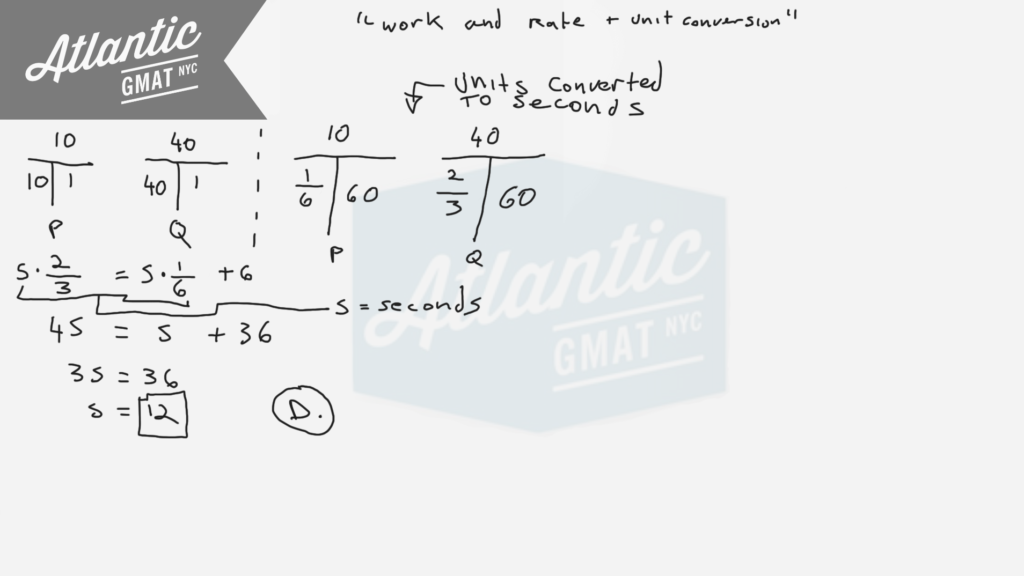Circular gears P and Q start rotating at the same time at constant speeds. Gear P makes 10 revolutions per minute and Gear Q makes 40 revolutions per minute. How many seconds after the gears start rotating will gear Q have made exactly 6 more revolutions than gear P?

(A) 6
(B) 8
(C) 10
(D) 12
(E) 15

Another question from the GMAT Prep software so avoid if you haven't yet worked through the free official practice tests so that you can keep those fresh. There's more work and rate practice below that you can safely work through now. This is technically a work and rate cooperative rate question even though it doesn't seem like it. I'd still use the T's to organize the information but then I'd probably move to algebraic translation. After setting up the first T's go ahead and do the unit conversion. We have to convert to seconds so multiply your minutes by 60 and then do the arithmetic for the rest of the chart. From there we need to figure out: How many seconds after the gears start rotating will gear Q have made exactly 6 more revolutions than gear P? So the seconds (S) in my equation are the same for gears P and Q because the gears are rotating at the same time. The only difference is that we need to add 6 revolutions to P's side the equation (to balance out the fact that Q will have made exactly 6 more revolutions than P).

Here are more Official GMAT distance, work, and rate question for which we use the T method:

Pumps A, B, and C operate at their respective constant rates

A company has two types of machines, type R and type S

A boat traveled upstream 90 miles at an average speed of (v-3) miles per hour and then traveled the same distance downstreamHere's another set of cooperative work and rate questions to practice on:

Work and Rate 1

Work and Rate 2

Work and Rate 3

Work and Rate 4

Work and Rate 5

For more distance, work, and rate examples you can also check out our GMAT Question of the Day page

CONTACT

Atlantic GMAT

405 East 51st St.

NY, NY 10022

(347) 669-3545

info@AtlanticGMAT.com